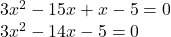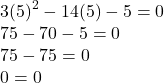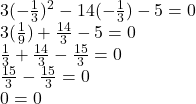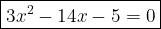## Write a quadratic equation given the roots -1/3 and 5, show your work

Question

Write a quadratic equation given the roots -1/3 and 5, show your work

in progress 0
6 months 2021-08-19T19:34:26+00:00 1 Answers 0 views 0

## Answers ( )

1.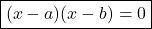The equation above is the intercept form. Both a-term and b-term are the roots of equation.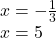These are the roots of equation. Therefore we substitute a = – 1/3 and b = 5 in the equation.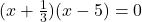Here we can convert the expression x+1/3 to this.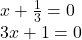Rewrite the equation.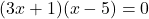Simplify by multiplying both expressions.